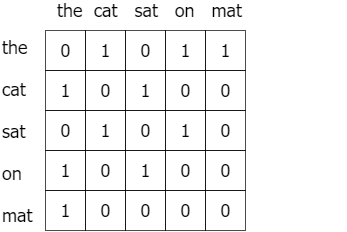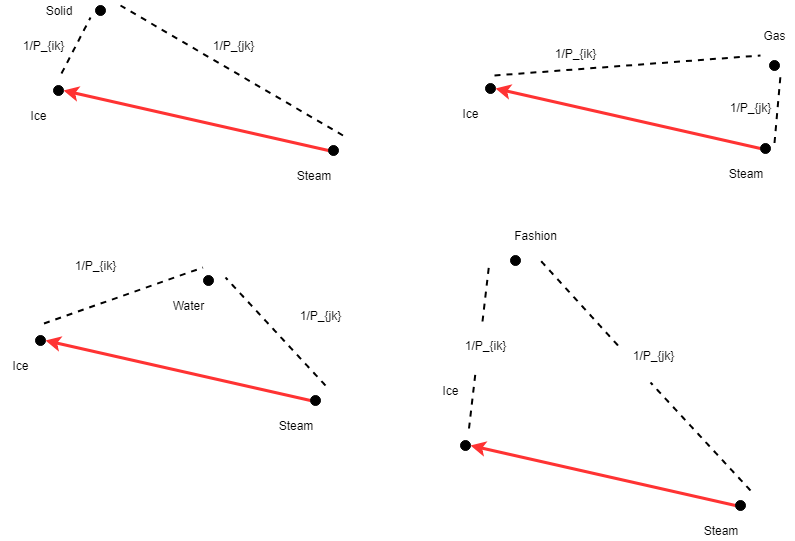###### Understanding Word2vec embedding with Tensorflow implementation
2022-05-09###### 23 Useful but less used Pandas Functions
2022-05-11

In this article, you will learn about GloVe, a very powerful word vector learning technique. This article will focus on explaining why GloVe is better and the motivation behind the cost function of GloVe which is the most crucial part of the algorithm. The code will be discussed in detail in a later article.

GloVe is a word vector technique that rode the wave of word vectors after a brief silence. Just to refresh, word vectors put words to a nice vector space, where similar words cluster together and different words repel. The advantage of GloVe is that, unlike Word2vec, GloVe does not rely just on local statistics (local context information of words), but incorporates global statistics (word co-occurrence) to obtain word vectors. But keep in mind that there’s quite a bit of synergy between the GloVe and Word2vec.

And don’t be surprised to hear that the idea of using global statistics to derive semantic relationships between words goes a long way back. Back to, latent semantic analysis (LSA). That’s just a fun fact. Let’s move on.

## Refresher on Word2vec

What’s the fundamental idea behind Word2vec?

You shall know a word by the company it keeps

J.R. Firth

Word vectors were built on this idea. Basically, you get a large corpus and make a dataset of tuples, where each tuple contains (some word x, a word in the context of x). Then you would use your old friend, a neural network, to learn to predict the context word of x, given the word x.

## So what’s pulling back?

Given the conspicuous performance of Word2vec, why not stick with it? The reason doesn’t lie in performance, but in the fundamentals of the solution formulation. Remember that, Word2vec relies only on local information of language. That is, the semantics learned for a given word, are only affected by the surrounding words.

For example, take the sentence: “The cat sat on the mat”

If you use Word2vec, it wouldn’t capture information like: Is “the” a special context of the words “cat” and “mat” ? or Is “the” just a stopword?

This can be suboptimal, especially in the eye of theoreticians.

## Enter, GloVe.

GloVe stands for “Global Vectors”. And as mentioned earlier, GloVe captures both global statistics and local statistics of a corpus, in order to come up with word vectors. But do we need both global and local statistics?

## Is two better than one?

Turns out, that each type of statistic has its own advantage. For example, Word2vec which captures local statistics does very well in analogy tasks. However, a method like LSA which uses only global statistics does not do that well in analogy tasks. However, since Word2vec method suffers from certain limitations (like what we discussed above) due to using local statistics only.

## Introduction to GloVe

GloVe method is built on an important idea,

You can derive semantic relationships between words from the co-occurrence matrix.

Given a corpus having V words, the co-occurrence matrix X will be a V x V matrix, where the i th row and j th column of XX_ij denotes how many times word i has co-occurred with word j. An example co-occurrence matrix might look as follows.The co-occurrence matrix for the sentence “the cat sat on the mat” with a window size of 1. As you probably noticed it is a symmetric matrix.

How do we get a metric that measures semantic similarity between words from this? For that, you will need three words at a time. Let me concretely lay down this statement.

Consider the entity

P_ik/P_jk where P_ik = X_ik/X_i

Here P_ik denotes the probability of seeing word i and k together, which is computed by dividing the number of times i and k appeared together (X_ik) by the total number of times word i appeared in the corpus (X_i).

You can see that given two words, i.e. ice and steam, if the third word k (also called the “probe word”),

• is very similar to ice but irrelevant to steam (e.g. k=solid), P_ik/P_jk will be very high (>1),
• is very similar to steam but irrelevant to ice (e.g. k=gas), P_ik/P_jk will be very small (<1),
• is related or unrelated to either words, then P_ik/P_jk will be close to 1

So, if we can find a way to incorporate P_ik/P_jk to computing word vectors we will be achieving the goal of using global statistics when learning word vectors.

## From a metric to word vectors

If you enjoyed it so far, buckle up. It’s about to get rough! It is not very apparent how we can arrive at a word vector algorithm because,

• We don’t have an equation, e.g. F(i,j,k) = P_ik/P_jk, but just an expression.
• Word vectors are high-dimensional vectors, however, P_ik/P_jk is a scalar. So there’s a dimensional mismatch.
• There are three entities involved (i, j, and k). But computing loss function with three elements can get hairy, and needs to be reduced to two.

Answering these three questions is the main contribution of GloVe. Now let’s go through GloVe step by step and see how answering these three questions gives us a word vector algorithm.

I am using the following notation which is slightly different from the paper.

• wu — Two separate embedding layers
• w* — Transpose of w
• — co-occurrence matrix
• bw and bu — Biases of w and u respectively

### Let’s assume an equation

Answering the first question is easy. Just assume it. Assume that there is a function F which takes in word vectors of i,j and k which outputs the ratio we’re interested in.

F(w_i,w_j, u_k) = P_ik/P_jk

You should get a bit curious by now because we see two embedding layers playing (w and u). So why two? The paper says, often both these layers will perform equivalently and will only differ by the different random initialization. However, having two layers help the model to reduce overfitting.

Now back to the function. Word vectors are linear systems. For example, you can perform arithmetic in embedding space, e.g.

w_{king} — w_{male} + w_{female} = w_{queen}

Therefore, let’s change the above equation to the following,

F(w_i — w_j, u_k) = P_ik/P_jk

Why does w_i — w_j suit here? In fact, you can derive the nice properties you observe about P_ik/P_jk in the embedding space. Let me elaborate.Behavior of vector distances to a probe word w.r.t. w_i — w_j

So you can see how the distance (dashed line) changes as different words are considered. And the distance between two given words i and k, correlated with the reciprocal of the P_{ik}. And why was this the case? It is because we always computed distances w.r.t the word vector w_i — w_j (i.e. the red line). So it is not a bad idea to start with w_i — w_j.

## Vector to a scalar …

With one problem solved, we move on to the next. How do we make LHS a scalar? There’s a pretty straightforward answer to this. That is to introduce a transpose and a dot product between the two entities in the following way.

F((w_i — w_j)* . u_k) = P_ik/P_jk or,

If you assume a word vector as a Dx1 matrix, (w_i — w_j)* will be 1xD shaped which gives a scalar when multiplied with u_k.

## What can F be?

Next, if we assume F has a certain property (i.e. homomorphism between additive group and the multiplicative group) which gives,

F(w_i* u_k — w_j* u_k) = F(w_i* u_k)/F(w_j* u_k) = P_ik/P_jk

In other words this particular homomorphism ensures that the subtraction F(A-B) can also be represented as a division F(A)/F(B) and get the same result. And therefore,

F(w_i* u_k)/F(w_j* u_k) = P_ik/P_jk

and

F(w_i* u_k) = P_ik

## I pulled a little sneaky on ya…

Okay, I was being sneaky. Just because F(A)/F(B) = G(A)/G(B) you cannot say F(A) = G(A). Because F(A)/F(B)=2F(A)/2F(B), doesn’t mean F(A)=2F(A). And it is not clear (at least to me) from the original paper why this is assumed. But let me give you some intuition why this would be a safe assumption. If we are to define the above relationship correctly, it would be,

F(w_i* u_k) = c P_ik for some constant c

But with this, you also get F(w_j* u_k) = c P_jk for any j. So if the similarity between i and k grows by c, the similarity between j and k (for any j) will also grow by c. This means (in a way) that all word vectors will scale up/down by a factor c, which won’t do any harm as the relative topography is preserved.

Moving on, if we assume F=exp the above homomorphism property is satisfied. Then let us set,

Exp(w_i* u_k) = P_ik=X_ik/X_i

and

w_i* u_k = log(X_ik) — log(X_i)

Next, X_i is independent of k, we move log(X_i) to LHS,

w_i* u_k + log(X_i)= log(X_ik)

Now given that we do not have a bias yet in the equation, we’ll get a bit creative and express log(X_i) in neural network parlance we get,

w_i* u_k + bw_i +bu_k= log(X_ik)

or,

w_i* u_k + bw_i +bu_k — log(X_ik) = 0

where bw and bu are biases of the network.

## Defining a cost

In an ideal setting, where you have perfect word vectors, the above expression will be zero. In other words, that’s our goal or objective. So we will be setting the LHS expression as our cost function.

J(w_i, w_j)= (w_i* u_j + bw_i +bu_j — log(X_ij))²

Note that the square makes this a mean square cost function. No harm was done to the original findings. Also k has been replaced with j.

## The final cost function

But your work does not stop here, you still have to patch up an important theoretical problem. Ponder what would happen if X_ik = 0. If you kick off a little experiment with the above cost function, you will be seeing the most hated 3 letters for an ML practitioner, i.e. NaN. Because log(0) is undefined. The easy fix would be to use log(1+X_ik) known as Laplacian smoothing. But the luminaries behind the GloVe paper propose a sleeker way of doing this. That is to introduce a weighting function.

J = f(X_ij)(w_i^T u_j + bw_i +bu_j — log(X_ij))²

where f(X_ij) = (x/x_{max})^a if x < x_{max} else 0

## Conclusion

That wraps everything. GloVe is a word vector technique that leverages both global and local statistics of a corpus in order to come up with a principled loss function that uses both these. GloVe does this by solving three important problems.

• We don’t have an equation, e.g. F(i,j,k) = P_ik/P_jk, but just an expression (i.e. P_ik/P_jk).
• Word vectors are high-dimensional vectors, however, P_ik/P_jk is a scalar. So there’s a dimensional mismatch.
• There are three entities involved (i, j, and k). But computing loss function with three elements can get hairy, and needs to be reduced to two.

The code for implementing GloVe with Keras is provided [here]

Source:

https://towardsdatascience.com/light-on-math-ml-intuitive-guide-to-understanding-glove-embeddings-b13b4f19c010##### Amir Masoud Sefidian
Machine Learning Engineer